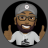#### Branch

##### Member
VIP
I would some to have a Bubble on my 10SMA. When the 10sma rise or fall the bubble rise and fall so that I can see it on my chart; not just the line but line and bubble.

T

#### tomsk

##### Well-known member
VIP
@Branch Here is your study as requested

Code:
``````# SMA Chart Bubble
# tomsk
# 1.14.2020

plot sma = Average(close, 10);
def Data = HighestAll(if isNaN(close[-1]) then sma else Double.NaN);

AddchartBubble(isNaN(close) and !isNaN(close), data, "SMA(10) at \n" + Round(data,2), Color.Yellow);
# End SMA Chart Bubble``````

•BenTen#### Branch

##### Member
VIP
@tomsk - OMG you and BenTen are the masters.
I have been up all night trying to transpose this code (see below) to do what you provided above.
QQ: That "\n" in your code (divide by) what does the "n" represent?

input barsBack = 0;
input price= close;

def vClose = close;
def nan = double.NaN;
def bn = BarNumber();
def month = GetMonth();
def monthDay = GetDayOfMonth(GetYYYYMMDD());
def currentBar = HighestAll(if !IsNaN(price) then bn else Double.NaN);
def highestClose = HighestAll(if IsNaN(vClose[-1]) then vClose else nan);
plot hc = highestClose;
hc.SetPaintingStrategy(PaintingStrategy.DASHES);
hc.SetDefaultColor(Color.DARK_ORANGE);

# throws an error symbol at top left of chart when condition is false. click on the error symbol to view the error message
# condition , "text"
assert(barsBack >= 0, "''bars back'' cannot be negative ");
# variables

AddChartBubble(bn == currentBar - barsBack, price,
# This section for text
"Price " + ( if price == close then "\$ " else if price == open then "Open: \$" else if price == low then " Low: \$" else if price == high then " High: \$" else if price == OHLC4 then " OHLC4 \$" else if price == HLC3 then " HLC3 \$" else " Value: " )+ price,(

# this section for color
if price == close then color.cyan else if price == open then color.pink else if price == low then color.yellow else if price == high then Color.White
else if price == OHLC4 then Color.Dark_Orange else if price == HLC3 then Color.Magenta else color.Plum), yes);#### BenTen

Staff
VIP
@Branch Thanks for the love. It was all @tomsk on this one#### Branch

##### Member
VIP
Okay @tomsk one last favor. Can you add a horizontal line (left to right) for the SMA price bubble? After this, I believe I would have my set-up (system for trading) in place.

T

#### tomsk

##### Well-known member
VIP
@tomsk - OMG you and BenTen are the masters.
I have been up all night trying to transpose this code (see below) to do what you provided above.
QQ: That "\n" in your code (divide by) what does the "n" represent?

Very observant, all the "\n" in my code above is to force a new line to be inserted at that point. Try removing that "\n" and see the effects of what the resulting output would looks like. This by the way only works on bubbles, you can't use this for labels.

Okay @tomsk one last favor. Can you add a horizontal line (left to right) for the SMA price bubble? After this, I believe I would have my set-up (system for trading) in place.

Per your request, here is a horizontal line plotted across the chart at the current value of the SMA(10)

Code:
``````# SMA Chart Bubble with Horizontal Line
# tomsk
# 1.14.2020

def sma = Average(close, 10);
def Data = HighestAll(if isNaN(close[-1]) then sma else Double.NaN);
plot hLine = HighestAll(if isNaN(close[-1]) then sma else Double.NaN);
AddchartBubble(isNaN(close) and !isNaN(close), data, "SMA(10) at \n" + Round(data,2), Color.Yellow);
# End SMA Chart Bubble``````#### Branch

##### Member
VIP
Thanks Tomsk
I worked on it this early this morning and figured it out; I came up with this on the 20ma and 10ma: (Again thank you tomsk)

input barsBack = 0;
input price = close;
input length1 = 20;
input displace = 0;
input showBreakoutSignals = no;

#---------------------------------------------------------------------
plot MA1 = Average(price[-displace], length1);

#---------------------------------------------------------------------
plot UpSignal1 = price crosses above MA1;
plot DownSignal1 = price crosses below MA1;

# MovingAverage--------------------------------------------------------------------
UpSignal1.SetHiding(!showBreakoutSignals);
DownSignal1.SetHiding(!showBreakoutSignals);

MA1.SetDefaultColor(GetColor(1));

def Data1 = HighestAll(if IsNaN(close[-1]) then MA1 else Double.NaN);

AddChartBubble(IsNaN(close) and !IsNaN(close), Data1, "20 Moving Avg \n" , Color.YELLOW);

#----------------------------------------------------------------------------------------------------
def nan = double.NaN;
def bn = BarNumber();
def currentBar = HighestAll(if !IsNaN(price) then bn else Double.NaN);
def MA1Close = HighestAll(if IsNaN(close[-1]) then close else nan);
plot DATA = DATA1;

Last edited:Can you add "AddChartBubble"to a Lower Chart? Questions 2AddChartBubble at a specific time Questions 3AddChartBubble when condition is met Questions 0